## Use self-similarity to get a limit from an inferior or superior limit.

### Quick description

In some situations the existence of a limit can be derived by using inferior or superior limit and suitably dividing a domain to use self-similarity.

### Prerequisites

Basic real analysis.

### Example 1: Maximal density of a packing

Fix a compact domain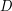in Euclidean space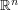(for example, a ball). A packing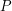is then a union of domains congruents to, with disjoint interiors. The density of a packing is defined as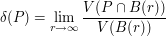where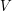is the volume and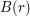is the square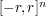, when the limit exists.

The present trick shows that if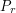is a packing contained inof maximal volume, then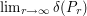exists.

First, since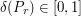, the superior limit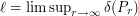exists. We want to prove that the inferior limit equals the superior one. Given any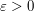, there is an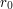such that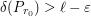. Now for any integer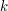the square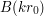can be divided into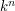translates of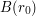. Each of these contains a packing of density greater than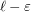, so that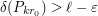for all. This rewrites as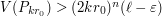. By maximality,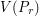is non-decreasing in; for all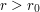one can introduce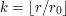and gets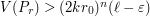. It follows that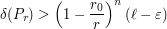so that as soon asis large enough,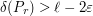. As a consequence,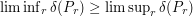and we are done.

### Example 2: Weyl's inequality and polynomial equidistribution

This example is taken from a mathoverflow question and answer.

Let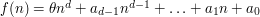be a polynomial with real coefficients, andirrational. Let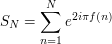Weyl's Equidistribution theorem for polynomials is equivalent to the claim that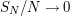as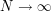. Though it is not the most easy way to prove this, let us deduce this theorem from the following Weyl's inequality.

Let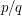be a rational number in lowest terms with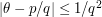. Weyl's Inequality is the bound: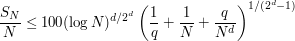Ifand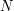are both large enough, and of the same order of magnitude, then the right-hand side gets small. The point is that the conditions onprevent one to apply this to arbitrary. However, Dirichlet's theorem tells us that arbitrary highsatisfy the needed condition, so that Weyl's inequality implies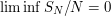.

Now the trick comes into play: the right-hand side in Weyl's inequality does not depend on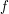, but only on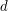. It therefore gives a uniform bound simultaneously for the sum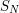and the sums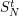computed using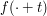instead of.

For all, there is therefore a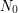such that for all,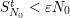. Then given any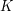, one has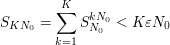Then, for all, letting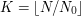we get that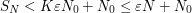. It follows that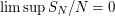.

### General discussion

The principle of the trick is the following: dividing the domain (either geometric in optimization setting, of the domain of sumation or integration) can allow you to take an estimate on your sequence that holds at a point, and propagate it to all its multiples. Then, for all other terms you usually get a bounded additional error term, which is hopefully negligible. This can especially be applied when a compactness argument provides you with an inferior or superior limit.